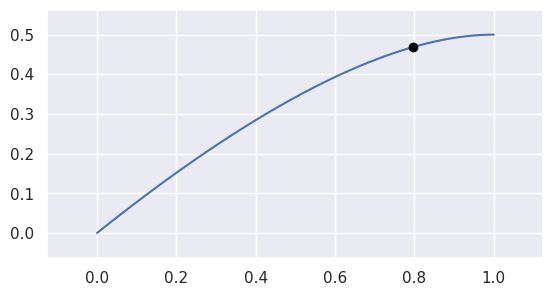# 貝茲曲線 Bézier curve

Max預期要移到新的坐標是 [880,738] ，可是Max自己亂寫的code 會判斷為 [878,199] 就是上圖畫面中正間的橘點，是錯的非常離譜，但自己動腦去寫又覺得很難，感謝已經有好心人幫忙寫出實用的工具。

## 工具下載：

github:
https://github.com/dhermes/bezier

## 安裝：

The `bezier` Python package can be installed with pip:

```\$ python -m pip install --upgrade bezier
\$ python3 -m pip install --upgrade bezier
\$ python -m pip install --upgrade bezier[full]```

## 解決 bezier 無法在 python3.9 安裝的問題

```BEZIER_NO_EXTENSION=true \
python3 -m pip install --upgrade bezier --no-binary=bezier```

## Why Bézier?

A Bézier curve (and triangle, etc.) is a parametric curve that uses the Bernstein basis:

to define a curve as a linear combination:

This comes from the fact that the weights sum to one:

This can be generalized to higher order by considering three, four, etc. non-negative weights that sum to one (in the above we have the two non-negative weights `s` and `1 - s`).

Due to their simple form, Bézier curves:

• can easily model geometric objects as parametric curves, triangles, etc.
• can be computed in an efficient and numerically stable way via de Casteljau’s algorithm
• can utilize convex optimization techniques for many algorithms (such as curve-curve intersection), since curves (and triangles, etc.) are convex combinations of the basis

Many applications — as well as the history of their development — are described in “The Bernstein polynomial basis: A centennial retrospective“, for example;

• aids physical analysis using finite element methods (FEM) on isogeometric models by using geometric shape functions called NURBS to represent data
• used in robust control of dynamic systems; utilizes convexity to create a hull of curves

## Getting Started

For example, to create a curve:

```>>> nodes1 = np.asfortranarray([
...     [0.0, 0.5, 1.0],
...     [0.0, 1.0, 0.0],
... ])
>>> curve1 = bezier.Curve(nodes1, degree=2)```

The intersection (points) between two curves can also be determined:

```>>> nodes2 = np.asfortranarray([
...     [0.0, 0.25,  0.5, 0.75, 1.0],
...     [0.0, 2.0 , -2.0, 2.0 , 0.0],
... ])
>>> curve2 = bezier.Curve.from_nodes(nodes2)
>>> intersections = curve1.intersect(curve2)
>>> intersections
array([[0.31101776, 0.68898224, 0. , 1. ],
[0.31101776, 0.68898224, 0. , 1. ]])
>>> s_vals = np.asfortranarray(intersections[0, :])
>>> points = curve1.evaluate_multi(s_vals)
>>> points
array([[0.31101776, 0.68898224, 0. , 1. ],
[0.42857143, 0.42857143, 0. , 0. ]])```

and then we can plot these curves (along with their intersections):

```>>> import seaborn
>>> seaborn.set()
>>>
>>> ax = curve1.plot(num_pts=256)
>>> _ = curve2.plot(num_pts=256, ax=ax)
>>> lines = ax.plot(
...     points[0, :], points[1, :],
...     marker="o", linestyle="None", color="black")
>>> _ = ax.axis("scaled")
>>> _ = ax.set_xlim(-0.125, 1.125)
>>> _ = ax.set_ylim(-0.0625, 0.625)```

For API-level documentation, check out the Bézier Python package documentation.

## Development

To work on adding a feature or to run the functional tests, see the DEVELOPMENT doc for more information on how to get started.

## Citation

For publications that use `bezier`, there is a JOSS paper that can be cited. The following BibTeX entry can be used:

```@article{Hermes2017,
doi = {10.21105/joss.00267},
url = {https://doi.org/10.21105%2Fjoss.00267},
year = {2017},
month = {Aug},
publisher = {The Open Journal},
volume = {2},
number = {16},
pages = {267},
author = {Danny Hermes},
title = {Helper for B{\'{e}}zier Curves, Triangles, and Higher Order Objects},
journal = {The Journal of Open Source Software}
}```

particular version of this library can be cited via a Zenodo DOI; see a full list by version.

Max 只用到2個函數分別是 evaluate() 和 locate().

`evaluate`(s)

Evaluate 𝐵(𝑠)B(s) along the curve.

This method acts as a (partial) inverse to `locate()`.

See `evaluate_multi()` for more details.```>>> nodes = np.asfortranarray([
...     [0.0, 0.625, 1.0],
...     [0.0, 0.5  , 0.5],
... ])
>>> curve = bezier.Curve(nodes, degree=2)
>>> curve.evaluate(0.75)
array([[0.796875],
[0.46875 ]])
```

Parameters

s (float) – Parameter along the curve.Returns

The point on the curve (as a two dimensional NumPy array with a single column).Return type

numpy.ndarray

`locate`(point)

Find a point on the current curve.

Solves for 𝑠s in 𝐵(𝑠)=𝑝B(s)=p.

This method acts as a (partial) inverse to `evaluate()`.

Note

A unique solution is only guaranteed if the current curve has no self-intersections. This code assumes, but doesn’t check, that this is true.```>>> nodes = np.asfortranarray([
...     [0.0, -1.0, 1.0, -0.75 ],
...     [2.0,  0.0, 1.0,  1.625],
... ])
>>> curve = bezier.Curve(nodes, degree=3)
>>> point1 = np.asfortranarray([
...     [-0.09375 ],
...     [ 0.828125],
... ])
>>> curve.locate(point1)
0.5
>>> point2 = np.asfortranarray([
...     [0.0],
...     [1.5],
... ])
>>> curve.locate(point2) is None
True
>>> point3 = np.asfortranarray([
...     [-0.25 ],
...     [ 1.375],
... ])
>>> curve.locate(point3) is None
Traceback (most recent call last):
...
ValueError: Parameters not close enough to one another
```

Parameters

point (numpy.ndarray) – A (`D x 1`) point on the curve, where 𝐷D is the dimension of the curve.Returns

The parameter value (𝑠s) corresponding to `point` or `None` if the point is not on the `curve`.Return type

ValueError – If the dimension of the `point` doesn’t match the dimension of the current curve.

```distance = spline_util.get_distance(point1,point1,point2,point2)
round_offset = 33
round_offset_rate = round_offset / distance
print('distance:',distance)
print('round_offset:',round_offset)
print('round_offset_rate:',round_offset_rate)
print(curve.evaluate(round_offset_rate))```